## A Probabilistic Derivation of the Linear Kalman Filter

Introduction

The Kalman Filter is one of those things – it doesn’t make any sense until you understand it. Then once you understand it, you don’t remember what was difficult. It took me an embarrassingly long time to get a grip on it, and a big part of that is that there are a lot of resources that provide a little bit of information, each with slightly different notation, so I’ll add one more to the pile. This will cover the probabilistic derivation of the Kalman Filter, with no example. I may add an example in a later post.

The Kalman Filter uses measurements and a guess from the previous state to tell us what the state of the system PROBABLY is. It also tells you how much to trust your estimate. How does it do this? By (not-correctly-but-close-enough) assuming that our variables are multivariate Gaussian distribution, and our noise is zero mean Gaussian and doesn’t covary with our states. If you aren’t familiar with Gaussian distributions, you just need to know that they have some very nice mathematical properties, which is why we use them even if they don’t perfectly describe the underlying process. This derivation is built on two properties of Gaussians which show how to condition Gaussian random vector x on Gaussian random vector y.

(1)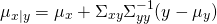(2)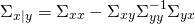To round out our background knowledge and system description, the equations that describe the system are:

(3)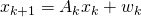(4)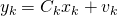Depending of what you’ve been reading, you may note that there is no control term (commonly denoted) in 3. I’ve left it off for simplicity, but if you understand the derivation well it’s straightforward to add.

Variables

Now that I’ve introduced a little bit of math, I’m going to outline all of the variables that get used. One of the things I’ve found most confusing about the Kalman filter is how many variables there are flying around, so you may find yourself referring back to this quite a bit.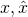– state and estimate of the state, respectively– a linear transform that uses the old state to find the new state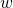– state update noise, which we assume to be zero mean Gaussian and uncorrelated to the state.– covariance of the state update noise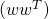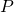– covariance of the state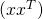– Kalman gain. For the purpose of this derivation, we can think of the Kalman gain as a notational convenience. This stackexchange post has an interesting perspective on it, and it also appears (via a completely different derivation) in the Minimum Variance Unbiased Estimator.– our measurement– describes the relationship between our measurement and the state. (See 4).– measurement noise, which we assume to be zero mean Gaussian and uncorrelated to the measurement.– covariance of the measurement noise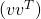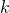– index variable, which increments by 1 at each reading.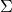– denotes a covariance matrix between its subscripted variables.

Derivation

Recall from the introduction, our goal is to figure out what our state,, is, given our measurements,. If we refer back to 1 and 2, we can see that the problem comes down to filling in the variables of our distribution:(Which is math notation for the multivariate normal, with the expected values to the left, and covariances to the right).

We designate ouras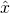(because), and use 4 to find our expected value of y, based on x. Note that throughout this section I’ve dropped the variable indices for neatness.

(5)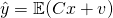Using the facts that expected value is a linear operator, C is a known, and v is assumed zero mean Gaussian, we can say:

(6)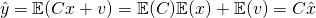Now we need to find the covariances. By definition:

(7)For the other covariances, we note that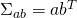.

(8)We note the definition of Q and P, and also that the noise is assumed not to covary with the state.

(9)Great! Two down, two to go. These are quick – remember that v and x are assumed independent (i.e., they do not covary).

(10)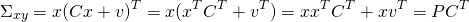(11)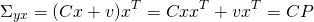Now we have the matrices describing our distribution:

(12)And we can apply 1 and 2 to find our updates:

(13)(14)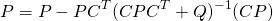.

Remember when I mentioned K just being a notational convenience? Notice the similarities in the previous two equations? The Kalman gain,is simply the common term in these two equations. I’m also going to (clumsily) re-introduce the indices, to give us our Measurement Update Equations.

(15)(16)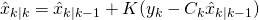(17)So why the subscriptsand? In short, we have some knowledge of what we expect the state to be at the new time, and we don’t want to just throw that useful information away. So what we do is update the expected values ofandbased on our model in the Prediction Step. This means that the information coming in from our measurements doesn’t need to try to compensate for the change in state, it just adjusts the error in the state update. Variables based on the prediction step are reliant on the previous measurement, and are thus denotedwhile variables based on the measurement update step are reliant only on estimates made at the current timestep, and are thus denoted. Rememberrepresents the current time, so sayingmeans “everything we know about x from information available at the current time” andmeans “everything we know about x from information available at the last timestep.”

So how do we perform the prediction step? First, we use our model to estimate x at the next timestep. This is the most straightforward part of the whole algorithm, though since it concerns a transition between states, you might see variation in whether it’s denotedor.

(18)And for P:

(19)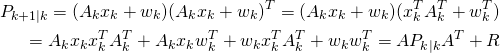And that ends the derivation.

Summary and Equations

This derivation shows the Kalman filter as an exploitation of the rules of Gaussians. When it comes down to it, the tasks is just to find the information needed to perform the conditioning operation, as shown in 1 and 2. Of course, this is only one derivation of one kind of Kalman Filter. For nonlinear systems, there is the Extended Kalman Filter, Unscented Kalman Filter, and others.

Measurement Update Step

(20)(21)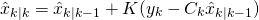(22)Prediction Step

(23)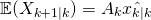(24)